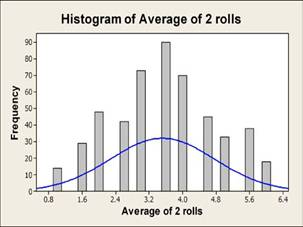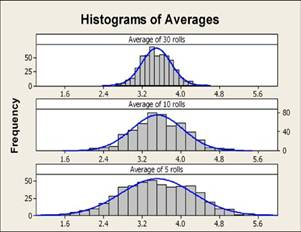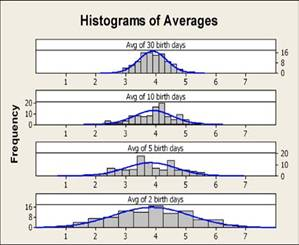#Quality Digest

Featured Product
This Week in Quality Digest Live
Six Sigma Features
Richard Harpster
Good news? You are probably already doing it.
Donald J. Wheeler
Does your approach do what you need?
James J. Kline
Quality professional organizations need to adjust their body of knowledge to include an understanding of big data
Donald J. Wheeler
In spite of what everyone says to the contrary
Brittney McIver
Every CAPA should begin with investigation
Six Sigma News
Elsmar Cove is a leading forum for quality and standards compliance
Is the future of quality management actually business management?
Too often process enhancements occur in silos where there is little positive impact on the big picture
Collect measurements, visual defect information, simple Go/No-Go situations from any online device
Good quality is adding an average of 11 percent to organizations’ revenue growth
Floor symbols and decals create a SMART floor environment, adding visual organization to any environment
A guide for practitioners and managers
Making lean Six Sigma easier and adaptable to current workplaces
Gain visibility into real-time quality data to improve manufacturing process efficiency, quality, and profits
Six Sigma

## Understanding the Central Limit Theorem

### Tumbling dice and birthdays

Published: Wednesday, August 26, 2009 - 05:00Michelle ParetEston Martz

Story update 8/27/2009: An error was spotted and corrected by author in paragraph starting with "The population mean for a six-sided die..."

Mark Twain famously quipped that there were three ways to avoid telling the truth: lies, damned lies, and statistics. The joke works because statistics frequently seem like a black box—it can be difficult to understand how statistical theorems make it possible to draw conclusions from data that, on their face, defy easy analysis.

But because data analysis plays a critical role in everything from jet engine reliability to determining the shows we see on television, it’s important to acquire at least a basic understanding of statistics. One of the most important concepts to understand is the central limit theorem.

In this article, we will explain the central limit theorem and show how to demonstrate it using common examples, including the roll of a die and the birthdays of Major League Baseball players.

### Defining the central limit theorem

A typical textbook definition of the central limit theorem goes something like this:

As the sample size increases, the sampling distribution of the mean, X-bar, can be approximated by a normal distribution with mean µ and standard deviation σ/√n where:

µ is the population mean
σ is the population standard deviation
n is the sample size

In other words, if we repeatedly take independent random samples of size n from any population, then when n is large, the distribution of the sample means will approach a normal distribution.

How large is large enough? Generally speaking, a sample size of 30 or more is considered to be large enough for the central limit theorem to take effect. The closer the population distribution is to a normal distribution, the fewer samples needed to demonstrate the theorem. Populations that are heavily skewed or have several modes may require larger sample sizes.

### Why does it matter?

The field of statistics is based upon the fact that it is rarely feasible or practical to collect all of the data from an entire population. Instead, we can gather a subset of data from a population, and then use statistics for that sample to draw conclusions about the population.

For example, we can collect random samples from an industrial process, then use the means of our samples to make conclusions about the stability of the overall process.

Two common characteristics used to define a population are the mean and standard deviation. When data follow a normal distribution, the mean indicates where the center of that distribution is, and the standard deviation reveals the spread.

Imagine you are getting the results of a test you took. In addition to receiving your own results, you also want to know your peers’ average score. However, if the test scores do not follow a normal distribution, the average could be misleading.

The central limit theorem is remarkable because it implies that, no matter what the population distribution looks like, the distribution of the sample means will approach a normal distribution. The theorem also allows us to make probability statements about the possible range of values the sample mean may take. This is because the normal distribution has a useful property called the empirical rule. The rule states that for data which follow a normal distribution:

68 percent of the data fall within 1σ of µ

95 percent of the data fall within 2σ of µ

99.7 percent of the data fall within 3σ of µ
95 percent of the data fall within 2σ of μ
99.7 percent of the data fall within 3σ of μ

### Watching the theorem work

Seeing how it can be applied makes the central limit theorem easier to understand, and we will demonstrate the theorem using dice and also using birthdays.

Example No. 1: Tumbling dice

Dice are ideal for illustrating the central limit theorem. If you roll a six-sided die, the probability of rolling a one is 1/6, a two is 1/6, a three is also 1/6, etc. The probability of the die landing on any one side is equal to the probability of landing on any of the other five sides.

In a classroom situation, we can carry out this experiment using an actual die. Alternatively, we can save time by using Minitab’s Calc > Random Data > Integer menu. To get an accurate representation of the population distribution, let’s roll the die 500 times. When we use a histogram to graph the data, we see that—as expected—the distribution looks fairly flat. It’s definitely not a normal distribution (figure 1).Figure 1: Because the odds of landing on all sides of a six-sided die are equal, the distribution of 500 die rolls is flat.

Let’s take more samples and see what happens to the histogram of the averages of those samples.

This time we will roll the die twice, and repeat this process 500 times. Again we can use Calc > Random Data > Integer to “roll” the die for us. We can then use Calc > Row Statistics to compute the average of each pair (figure 2).Figure 2: Minitab makes it easy to generate die-rolling data, then calculate the averages.

We can then create a histogram of these averages to view the shape of their distribution (figure 3). Although the blue normal curve does not accurately represent the histogram, the profile of the bars is looking more bell-shaped. Now let’s roll the die five times and compute the average of the five rolls, again repeated 500 times. Then, let’s repeat the process rolling the die 10 times, then 30 times.Figure 3: The distribution of 500 averages for two rolls of a die begins to resemble the familiar bell shape of a normal distribution.

The histograms for each set of averages (figure 4) show that as the sample size, or number of rolls, increases, the distribution of the averages comes closer to resembling a normal distribution. In addition, the variation of the sample means decreases as the sample size increases.Figure 4: As the number of rolls of the die increases, the distribution of averages approaches a normal distribution.

The central limit theorem states that for a large enough n, X-bar can be approximated by a normal distribution with mean µ and standard deviation σ/√n.

The population mean for a six-sided die is (1+2+3+4+5+6)/6 = 3.5 and the population standard deviation is 1.708. Thus, if the theorem holds true, the mean of the thirty averages should be about 3.5 with standard deviation 1.708/ 30 = 0.31. Using the dice we “rolled” using Minitab, the average of the thirty averages, depicted in Figure 4, is 3.49 and the standard deviation is 0.30, which are very close to the calculated approximations.

Example No. 2: Birthdays

Now let’s demonstrate the central limit theorem using birthdays. You’ll recall that the sides of dice have an equal probability. Contrary to popular belief, there is not necessarily an equal chance of being born on a Sunday instead of a Monday or any other day of the week. Currently, the most popular day for babies to be born in the United States is Wednesday—there are 15.4 percent more births on Wednesday than the average day; and from 1990 to 2006, Tuesday was the most popular birth day.

To demonstrate the central limit theorem using birthdays, we first need to collect some birth dates. Students could gather the birthdays of their friends, families, and colleagues. We will use the birthdays of the more than 700 Major League Baseball players, which are available on MLB.com.

Of course, most birthday information won’t include the day of the week, but using Minitab’s Data > Extract from Date/Time > To Numeric, we can easily find out which day each baseball player was born (figure 5). For example, Minitab can tell us that Derek Jeter, whose birthday is June 26, 1974, was born on a Wednesday.Figure 5: We can use Minitab to extract days of the week from our birthday data.

If we look at the histogram for the population of baseball players (figure 6), where No. 1 equals Sunday, No. 2 equals Monday, and so on, we can see that the birth days do not follow a normal distribution, and Tuesday (3) is the most popular day.Figure 6: This histogram shows that Tuesday is the most popular birth day for Major League Baseball players.

Just like we did in the dice experiment, we will now create samples of size two, randomly sampling two players, then another two, and so forth. Let’s take 100 samples total. To randomly sample players’ birthdays from the data in the worksheet, we can use Calc > Random Data > Sample From Columns.

Then let’s compute the average birth day for each sample of size two using Calc > Row Statistics.

We will repeat the random sampling and averaging for five players, then 10 players, then 30 players, and create histograms for each set of averages.

In the original histogram of more than 700 baseball players, we saw a non-normal distribution. When we look at the histograms of the averages (figure 7), we see they very quickly resemble a normal distribution and that the variation decreases as the sample size increases.Figure 7: The central limit theorem in action—as sample size increases, the distribution of the averages more closely resembles a normal distribution.

### Conclusion

The central limit theorem enables us to approximate the sampling distribution of X-bar with a normal distribution. This idea may not be frequently discussed outside of statistical circles, but it’s an important concept. We can make it easier to understand through simple demonstrations using dice, birthdays, dates on coins, airline flight delays, or cycle times.

With an improved understanding of the central limit theorem and other statistical concepts, students with eager minds will soon find it easier to distinguish between lies, damned lies, and the truth that lies behind good statistics.

This article was written by Michelle Paret, Product Marketing Manager, Minitab Inc. and Eston Martz Sr. Creative Services Specialist, Minitab Inc.

### Discuss